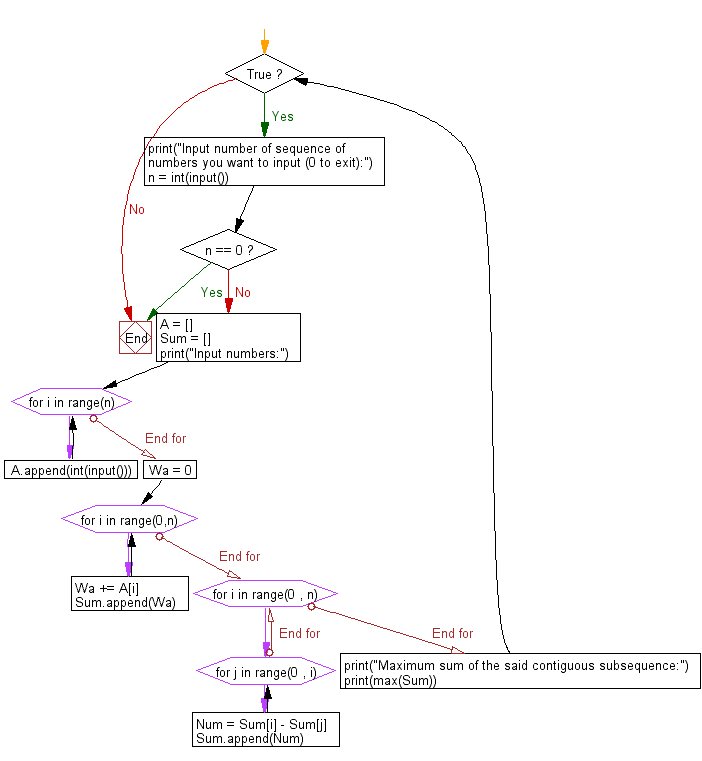﻿ Python: Find the maximum sum of a contiguous subsequence from a given sequence of numbers a1, a2, a3, ... an - w3resource# Python: Find the maximum sum of a contiguous subsequence from a given sequence of numbers a1, a2, a3, ... an

## Python Basic - 1: Exercise-44 with Solution

Write a Python program to find the maximum sum of a contiguous subsequence from a given sequence of numbers a1, a2, a3, ... an. A subsequence of one element is also a continuous subsequence.

Input:
You can assume that 1 ≤ n ≤ 5000 and -100000 ≤ ai ≤ 100000.
Input numbers are separated by a space.
Input 0 to exit.
Input number of sequence of numbers you want to input (0 to exit): 3
Input numbers:
2
4
6
Maximum sum of the said contiguous subsequence:
12 Input number of sequence of numbers you want to input (0 to exit): 0

Sample Solution:

Python Code:

``````while True:
print("Input number of sequence of numbers you want to input (0 to exit):")
n = int(input())
if n == 0:
break
else:
A = []
Sum = []
print("Input numbers:")
for i in range(n):
A.append(int(input()))
Wa = 0
for i in range(0,n):
Wa += A[i]
Sum.append(Wa)
for i in range(0 , n):
for j in range(0 , i):
Num = Sum[i] - Sum[j]
Sum.append(Num)
print("Maximum sum of the said contiguous subsequence:")
print(max(Sum))
``````

Sample Output:

```Input number of sequence of numbers you want to input (0 to exit):
3
Input numbers:
2
4
6
Maximum sum of the said contiguous subsequence:
12
Input number of sequence of numbers you want to input (0 to exit):
0
```

Flowchart:Python Code Editor:

Have another way to solve this solution? Contribute your code (and comments) through Disqus.

What is the difficulty level of this exercise?

Test your Programming skills with w3resource's quiz.

﻿

## Python: Tips of the Day

Iterating over dictionaries using 'for' loops:

I am a bit puzzled by the following code: d = {'x': 1, 'y': 2, 'z': 3} for key in d: print key, 'corresponds to', d[key] What I don't understand is the key portion. How does Python recognize ...

key is just a variable name.

```for key in d:
```

For Python 3.x:

```for key, value in d.items():
```

For Python 2.x:

```for key, value in d.iteritems():
```

To test for yourself, change the word key to poop.

In Python 3.x, iteritems() was replaced with simply items(), which returns a set-like view backed by the dict, like iteritems() but even better. This is also available in 2.7 as viewitems().

The operation items() will work for both 2 and 3, but in 2 it will return a list of the dictionary's (key, value) pairs, which will not reflect changes to the dict that happen after the items() call. If you want the 2.x behavior in 3.x, you can call list(d.items()).

Ref: https://bit.ly/37dm0Qo